Algorithms
Topics:
Graph Representation
• Searching
• Sorting
• Greedy Algorithms
• Graphs
• String Algorithms
• Dynamic Programming

# Graph Representation

Graphs are mathematical structures that represent pairwise relationships between objects. A graph is a flow structure that represents the relationship between various objects. It can be visualized by using the following two basic components:

• Nodes: These are the most important components in any graph. Nodes are entities whose relationships are expressed using edges. If a graph comprises 2 nodes $A$ and $B$ and an undirected edge between them, then it expresses a bi-directional relationship between the nodes and edge.

• Edges: Edges are the components that are used to represent the relationships between various nodes in a graph. An edge between two nodes expresses a one-way or two-way relationship between the nodes.

Types of nodes

• Root node: The root node is the ancestor of all other nodes in a graph. It does not have any ancestor. Each graph consists of exactly one root node. Generally, you must start traversing a graph from the root node.

• Leaf nodes: In a graph, leaf nodes represent the nodes that do not have any successors. These nodes only have ancestor nodes. They can have any number of incoming edges but they will not have any outgoing edges.

Types of graphs

• Undirected: An undirected graph is a graph in which all the edges are bi-directional i.e. the edges do not point in any specific direction.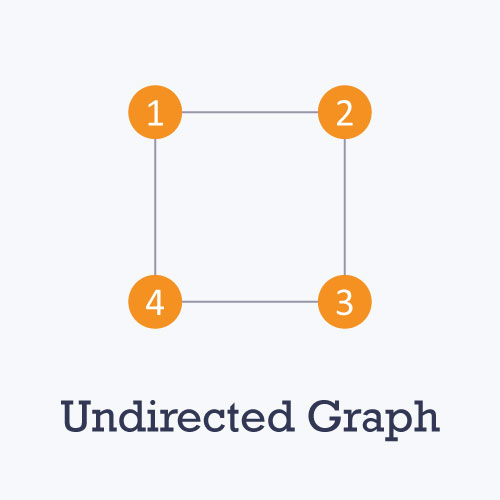• Directed: A directed graph is a graph in which all the edges are uni-directional i.e. the edges point in a single direction.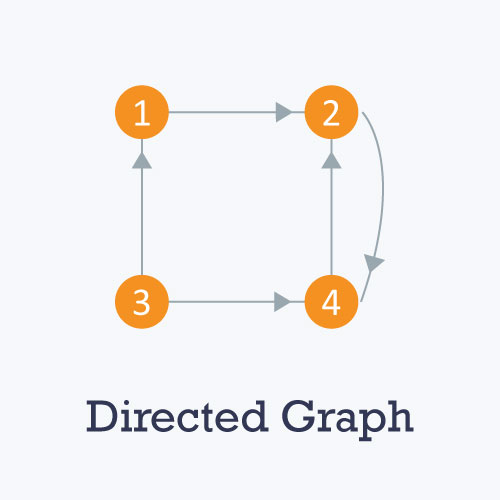• Weighted: In a weighted graph, each edge is assigned a weight or cost. Consider a graph of 4 nodes as in the diagram below. As you can see each edge has a weight/cost assigned to it. If you want to go from vertex 1 to vertex 3, you can take one of the following 3 paths:

• 1 -> 2 -> 3
• 1 -> 3
• 1 -> 4 -> 3

Therefore the total cost of each path will be as follows: - The total cost of 1 -> 2 -> 3 will be (1 + 2) i.e. 3 units - The total cost of 1 -> 3 will be 1 unit - The total cost of 1 -> 4 -> 3 will be (3 + 2) i.e. 5 units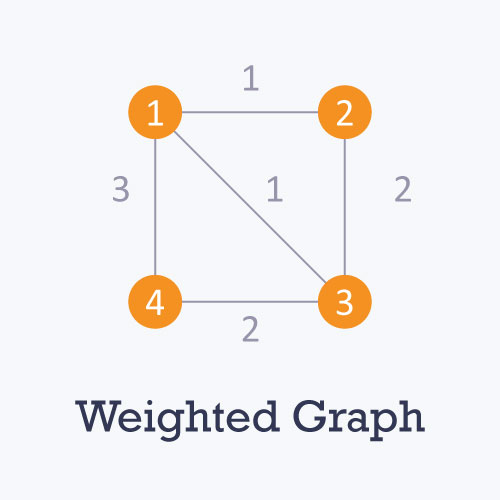• Cyclic: A graph is cyclic if the graph comprises a path that starts from a vertex and ends at the same vertex. That path is called a cycle. An acyclic graph is a graph that has no cycle.

A tree is an undirected graph in which any two vertices are connected by only one path. A tree is an acyclic graph and has N - 1 edges where N is the number of vertices. Each node in a graph may have one or multiple parent nodes. However, in a tree, each node (except the root node) comprises exactly one parent node.

Note: A root node has no parent.

A tree cannot contain any cycles or self loops, however, the same does not apply to graphs.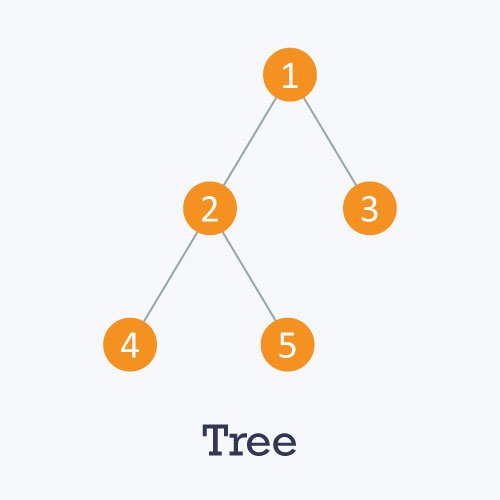Graph representation

You can represent a graph in many ways. The two most common ways of representing a graph is as follows:

An adjacency matrix is a VxV binary matrix A. Element $A_{i,j}$ is 1 if there is an edge from vertex i to vertex j else $A_{i,j}$ is 0.

Note: A binary matrix is a matrix in which the cells can have only one of two possible values - either a 0 or 1.

The adjacency matrix can also be modified for the weighted graph in which instead of storing 0 or 1 in $A_{i,j}$, the weight or cost of the edge will be stored.

In an undirected graph, if $A_{i,j}$ = 1, then $A_{j,i}$ = 1. In a directed graph, if $A_{i,j}$ = 1, then $A_{j,i}$ may or may not be 1.

Adjacency matrix provides constant time access (O(1) ) to determine if there is an edge between two nodes. Space complexity of the adjacency matrix is O($V^2$).

The adjacency matrix of the following graph is:
i/j : 1 2 3 4
1 : 0 1 0 1
2 : 1 0 1 0
3 : 0 1 0 1
4 : 1 0 1 0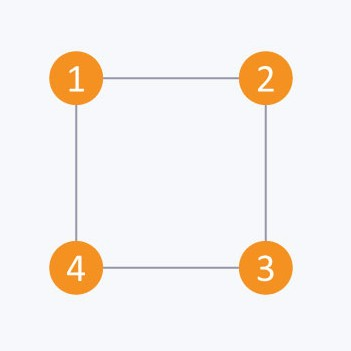The adjacency matrix of the following graph is:

i/j: 1 2 3 4
1 : 0 1 0 0
2 : 0 0 0 1
3 : 1 0 0 1
4 : 0 1 0 0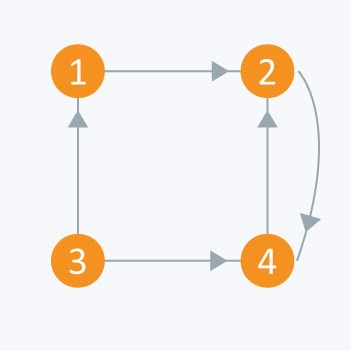Consider the directed graph given above. Let's create this graph using an adjacency matrix and then show all the edges that exist in the graph.

Input file
4 $\hspace{2cm}$ // nodes
5 $\hspace{2cm}$//edges
1 2 $\hspace{1.5cm}$ //showing edge from node 1 to node 2
2 4 $\hspace{1.5cm}$ //showing edge from node 2 to node 4
3 1 $\hspace{1.5cm}$ //showing edge from node 3 to node 1
3 4 $\hspace{1.5cm}$ //showing edge from node 3 to node 4
4 2 $\hspace{1.5cm}$ //showing edge from node 4 to node 2

Code

 #include <iostream>

using namespace std;

bool A;

void initialize()
{
for(int i = 0;i < 10;++i)
for(int j = 0;j < 10;++j)
A[i][j] = false;
}

int main()
{
int x, y, nodes, edges;
initialize();       //Since there is no edge initially
cin >> nodes;       //Number of nodes
cin >> edges;       //Number of edges
for(int i = 0;i < edges;++i)
{
cin >> x >> y;
A[x][y] = true;     //Mark the edges from vertex x to vertex y
}
if(A == true)
cout << “There is an edge between 3 and 4” << endl;
else
cout << “There is no edge between 3 and 4” << endl;

if(A == true)
cout << “There is an edge between 2 and 3” << endl;
else
cout << “There is no edge between 2 and 3” << endl;

return 0;
}


Output

There is an edge between 3 and 4.

There is no edge between 2 and 3.

The other way to represent a graph is by using an adjacency list. An adjacency list is an array A of separate lists. Each element of the array Ai is a list, which contains all the vertices that are adjacent to vertex i.

For a weighted graph, the weight or cost of the edge is stored along with the vertex in the list using pairs. In an undirected graph, if vertex j is in list $A_{i}$ then vertex i will be in list $A_{j}$.

The space complexity of adjacency list is O(V + E) because in an adjacency list information is stored only for those edges that actually exist in the graph. In a lot of cases, where a matrix is sparse using an adjacency matrix may not be very useful. This is because using an adjacency matrix will take up a lot of space where most of the elements will be 0, anyway. In such cases, using an adjacency list is better.

Note: A sparse matrix is a matrix in which most of the elements are zero, whereas a dense matrix is a matrix in which most of the elements are non-zero.Consider the same undirected graph from an adjacency matrix. The adjacency list of the graph is as follows:

A1 → 2 → 4
A2 → 1 → 3
A3 → 2 → 4
A4 → 1 → 3Consider the same directed graph from an adjacency matrix. The adjacency list of the graph is as follows:

A1 → 2
A2 → 4
A3 → 1 → 4
A4 → 2

Consider the directed graph given above. The code for this graph is as follows:

Input file

4 $\hspace{2cm}$ // nodes
5 $\hspace{2cm}$ //edges
1 2 $\hspace{1.5cm}$ //showing edge from node 1 to node 2
2 4 $\hspace{1.5cm}$ //showing edge from node 2 to node 4
3 1 $\hspace{1.5cm}$ //showing edge from node 3 to node 1
3 4 $\hspace{1.5cm}$ //showing edge from node 3 to node 4
4 2 $\hspace{1.5cm}$ //showing edge from node 4 to node 2

Code

 #include<iostream >
#include < vector >

using namespace std;

int main()
{
int x, y, nodes, edges;
cin >> nodes;       //Number of nodes
cin >> edges;       //Number of edges
for(int i = 0;i < edges;++i)
{
cin >> x >> y;
adj[x].push_back(y);        //Insert y in adjacency list of x
}
for(int i = 1;i <= nodes;++i)
{
cout << "Adjacency list of node " << i << ": ";
for(int j = 0;j < adj[i].size();++j)
{
if(j == adj[i].size() - 1)
cout << adj[i][j] << endl;
else
cout << adj[i][j] << " --> ";
}
}
return 0;
}


Output

• Adjacency list of node 1: 2
• Adjacency list of node 2: 4
• Adjacency list of node 3: 1 --> 4
• Adjacency list of node 4: 2

Try out this simple question:

Contributed by: Prateek Garg

?